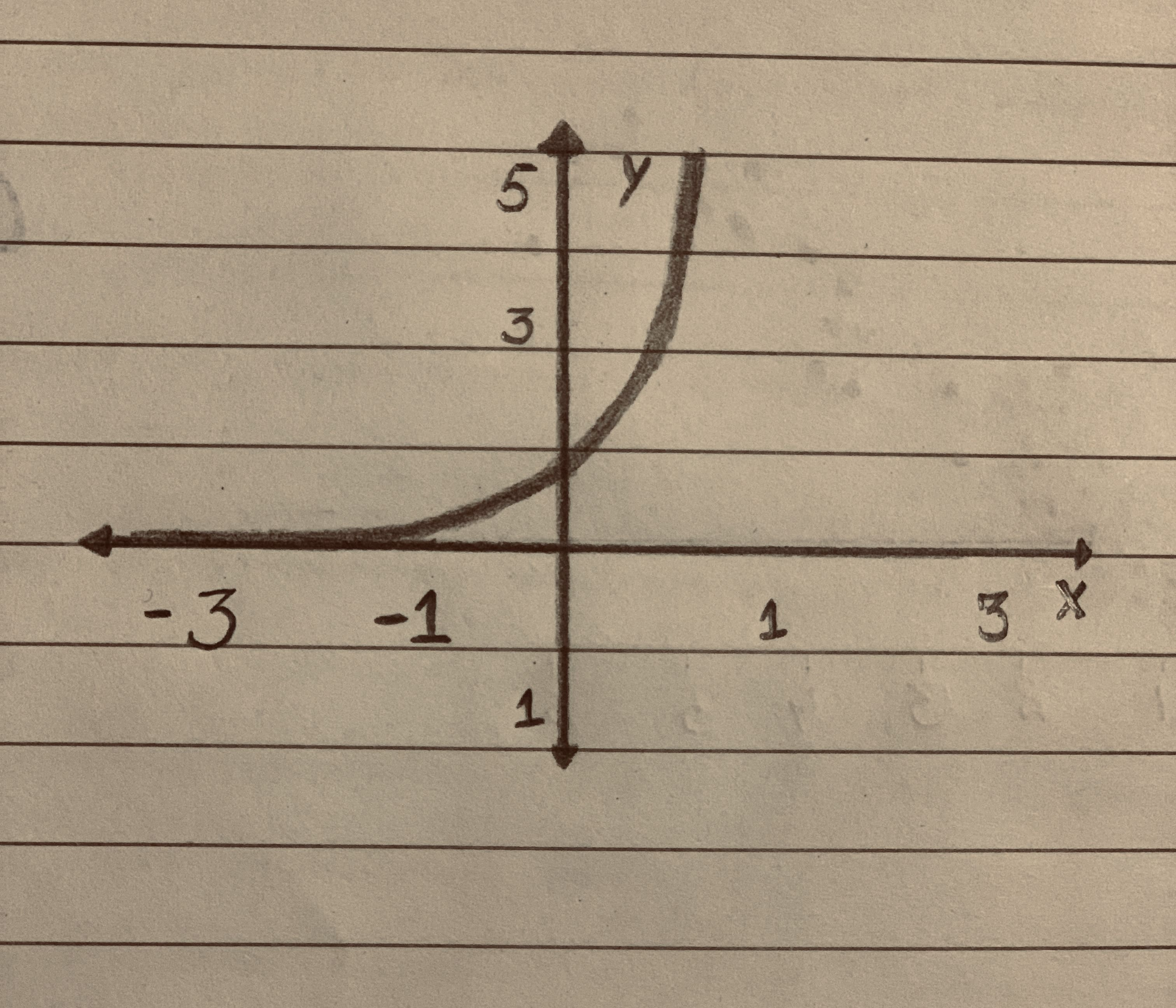# Exponential Growth & Decay Equations & Examples

Recent questions in Exponential growth and decayLennie Carroll 2020-12-05

## The equation $=y8000\left(1.09{\right)}^{x}$ describes the amount of an investment (in dollars) based on the number of years itsHaven 2020-12-05

## Determine whether the function represents exponential growth or exponential decay. Then identify the percent rate of change. $y=5\left(0.6{\right)}^{t}$Braxton Pugh 2020-12-05

## Determine of each of the following functions models exponential growth or decay $a\right)y={2}^{0.1x}$ $b\right)y={3}^{1.4x}$ $c\right)y=4{e}^{-5x}$ $d\right)y={0.8}^{3x}$facas9 2020-11-26

## The population (in millions) Ukraine in 2015 is 44.4 and the anticipated annualized rate of change over time k =−0.006. (a) Find the exponential growth model P = Cekt for the population by letting t = 5 correspond to 2015. (b) Use the model to project the country's population in 2030.(c) Discuss the relationship between the sign of k and the change in population for the country.ossidianaZ 2020-11-24

## In the exponential growth or decay function $y={y}_{0}{e}^{k}t$, what does ${y}_{0}$ represent? What does k represent?texelaare 2020-11-23

## Suppose that f is an exponential function with percentage growth rate of 5% and that f(0) = 5. Find a formula for f(x). f(x) =UkusakazaL 2020-11-20

## Determine the type of graph Increasing Linear, Decreasing Linear, Positive Quadratic, Negative Quadratic, Exponential Growth, or Exponential decay and give proof.Rui Baldwin 2020-11-16

## Perform the inverse transformation to express light intensity as an exponential function of depth in the lake. Display a scatterplot of the original data with exponential model superimposed. Is your exponential function a satisfactory model for the data?ruigE 2020-11-12

## Given the following exponential growth function, identify the growth rate in percentage form: Q=10,000⋅3.0T r=shadsiei 2020-11-11

## Identify the function as exponential growth or exponential decay.Then identify the growth or decay factor. $y=5.2\cdot {3}^{x}$rocedwrp 2020-11-08

## Polynomial function graphs have similarities depending on their degree. Explain how you can determine the best regression model by using finite differences. Then determine the end behavior of the graph based on the degree of a function and information gathered from a data table.tricotasu 2020-11-08

## Sasha drinks an energy beverage with caffeine. After ingesting the energizing beverage, Sashas's caffeine___?Nannie Mack 2020-11-08

## A district's population in 2005 was 28,300. With a continuous annual growth rate of approximately 7% what will the population be in 2020 according to the exponential growth function? To the nearest whole number, round the response.Suman Cole 2020-11-07

## For any elements a and to from a group and any integer n, prove that ${\left({a}^{-1}ba\right)}^{n}={a}^{-1}{b}^{n}a$.Armorikam 2020-10-23

## Determine whether each function represents exponential growth or decay. Write the base in terms of the rate of growth or decay, identify r, and interpret the rate of growth or decay. $y=450\cdot {2}^{x}$

Algebra IOpen questionsibuzwaW 2020-10-23

## The close connection between logarithm and exponential functions is used often by statisticians as they analyze patterns in data where the numbers range from very small to very large values. For example, the following table shows values that might occur as a bacteria population grows according to the exponential function P(t)=50(2t): Time t (in hours)012345678 Population P(t)501002004008001,6003,2006,40012,800 a. Complete another row of the table with values log (population) and identify the familiar function pattern illustrated by values in that row. b. Use your calculator to find log 2 and see how that value relates to the pattern you found in the log P(t) row of the data table. c. Suppose that you had a different set of experimental data that you suspected was an example of exponential growth or decay, and you produced a similar “third row” with values equal to the logarithms of the population data. How could you use the pattern in that “third row” to figure out the actual rule for the exponential growth or decay model?Harlen Pritchard 2020-10-21Harlen Pritchard 2020-10-18